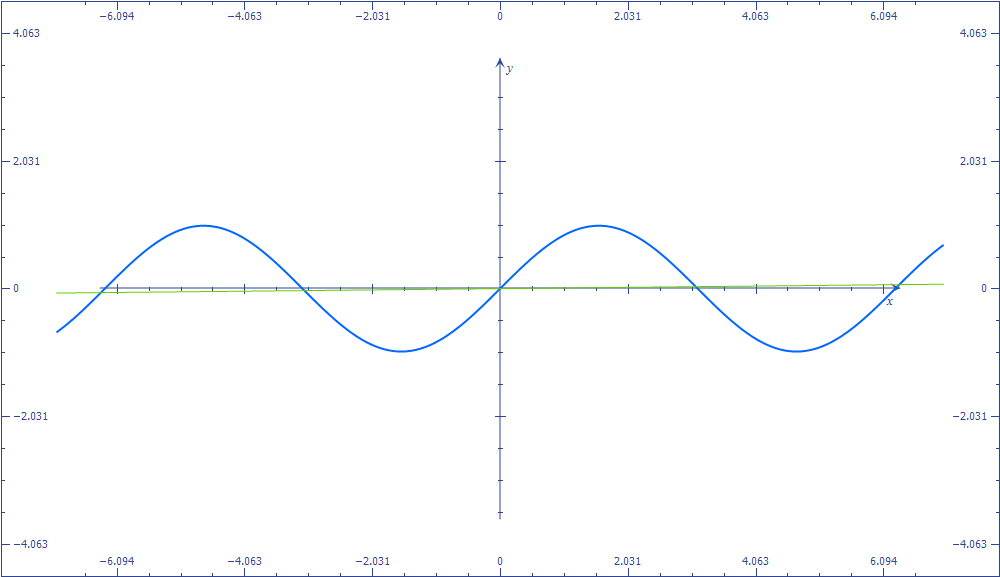# A Trigonometric Dilemma!

Geometry Level 5$\large{\sin(x) = \dfrac{x}{100}}$

Let $A$ be the number of real roots of the above equation if $x$ is measured in degrees.

Let $B$ be the number of real roots of the above equation if $x$ is measured in radians.

Find the value of $A+B$?

×

Problem Loading...

Note Loading...

Set Loading...